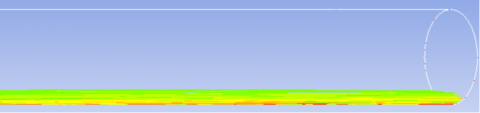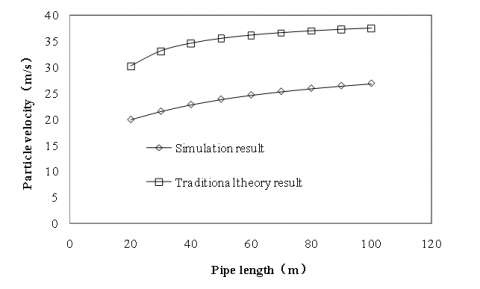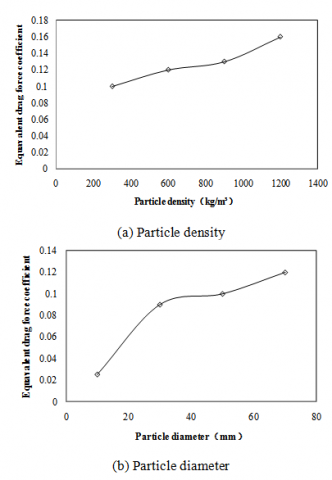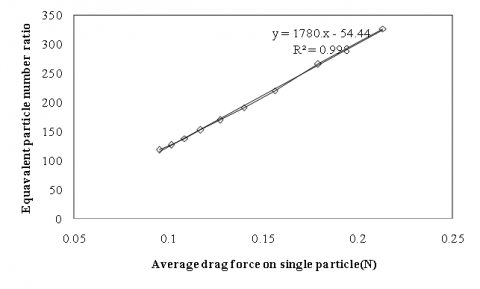# The simplified calculation model of pneumatic garbage transportation at acceleration period in horizontal straight pipe

The simplified calculation model of pneumatic garbage transportation at acceleration period in horizontal straight pipe

Zheng Li*Jia Li Wei Yang Jingbo Liang

College of Mechanical Engineering, Tianjin University, Tianjin 300072, China

Tianjin eco-city environmental protection Co. Ltd, Tianjin 300467, China

Corresponding Author Email:
lizheng0330@163.com
Page:
683-687
|
DOI:
https://doi.org/10.18280/ijht.350330
|
Accepted:
|
Published:
30 September 2017
| Citation

OPEN ACCESS

Abstract:

A numerical method has been used to analyze the pneumatic garbage transportation. The results show that the garbage made a bottom flow in horizontal straight pipe. A traditional pneumatic transportation empirical formula was used to get the velocity and pressure of pneumatic garbage transportation. The results of the empirical formula turned out to be vastly different from the simulation results, where the velocity error was 54.2% and the pressure gradient error was 134%. For the engineering application, a new theoretical model was proposed. The theoretical calculation results were compared with the simulation results, the velocity error was 2.4% and the additional pressure gradient error was 2.8%. In the new model, there were two key parameters. These were the equivalent drag coefficient and equivalent particle number ratio. The equivalent drag coefficient increased with enlargement of particle density and diameter and the equivalent particle number ratio increased linearly with an increase of average load on a single particle.

Keywords:

pneumatic garbage collection, horizontal straight pipe, simplified model, equivalent drag coefficient, equivalent particle number ratio

1. Introduction

Pneumatic refuse transportation was a clean and efficient waste collection technology [1-3]. It started in Sweden, where they used fans to generate high-speed airflow in buried pipes. The airflow conveyed waste to waste collection stations, where the garbage was separated from the airflow. At present, the traditional theory model of pneumatic transportation is mostly used in the design of the pneumatic refuse transportation system [4-5].

When the garbage ran through the horizontal straight pipe, it made acceleration initially, as the motive force was larger than the resistance. When the pipe was long enough, the force on the garbage would eventually reach equilibrium and then the velocity became consistent. This is the garbage movement state in the horizontal straight pipe. In the construction of pneumatic refuse transportation systems, the buried pipe needs to surround the municipal piping or some underground structures. There were few long straight pipes in the pipe network, which in turn caused the garbage to accelerate in horizontal straight pipe. The traditional pneumatic transportation theory model was used to get a characteristic parameter of garbage movement at an acceleration period in straight pipe. The results were compared with the simulation results and a new simplified model, based on the simulation data, was proposed.

2. Numerical Calculation

Pneumatic garbage transportation has developed over approximately 50 years and the parameters for this technology has been standardized. According to application experience in the field, the diameter of a pneumatic garbage transportation pipe was 500mm and air velocity, 40m/s. It was discovered that the air flow rate was 7.85m3/s. The pneumatic garbage transportation system collected the garbage from every single dustbin, every 5 seconds. The volume of one dustbin was 0.39m³ and any natural piled garbage porosity in the dustbin was between 40%~60% . Garbage porosity was defined as 50% in paper. The garbage volume flow rate was obtained at 0.039m3/s and the volume concentration of the solid phase was obtained at 0.5% in pneumatic garbage transportation. The Euler-Lagrange model was used in the numerical simulation [7-8].

The garbage was simplified as a ball particle in the paper. In the gas-solid two-phase flow, the solid particles bore gravity, drag force, Basset force and Saffman lift force etc. [9-10]. According to Newton's third law, the solid phase would generate an opposite reaction force on the fluid phase, which was the source item of the equilibrium equation (navier-stokes equations). The interaction between the fluid phase and the solid phase was taken into account in the paper.

The pipe model with a diameter of 0.5m and length of 100m, was set up in the paper to analyze the movement characteristics of garbage with different particle diameters (10mm, 30mm, 50mm, 70mm) and density (300kg/m³, 600kg/m³, 900kg/m³, 1200kg/m³) in the pipe. The boundary condition of velocity inlet and outflow outlet, was used for the fluid phase in the simulation model.

Figure 1 shows garbage particle distribution in the horizontal straight pipe when the garbage diameter was 10mm and the garbage velocity at pipe inlet was 0. The results show that garbage particles made bottom flow in horizontal straight pipe .

## 1.pngFigure 1. Contour of garbage movement in horizontalstraight pipe

3.1 Pressure model

According to the traditional pneumatic transportation theory, loss of pressure was caused by accelerating solid particles (as equation1) and the friction of gas and solid particles (as equation2) :

$\Delta P_{m a}=\frac{\frac{1}{2} G_{p}\left(u_{p 2}^{2}-u_{p 1}^{2}\right)}{A u_{a}}$     (1)

$\Delta P_{m f}=a \Delta P_{\alpha f}$     (2)

In equation1, △Pma was pressure caused by accelerating solid particles; Gp was solid particles mass flow rate; A was the area of pipe cross-section; ua was gas velocity and up1 and up2 were garbage inlet velocity and outlet velocity of the calculated pipeline.

In equation 2, △Pmf was the sum of pressure loss, caused by friction of gas and solid particles movement separately; △Paf was pressure loss, caused by gas friction and a was the ratio of pressure loss, caused by solid particles friction to pressure loss, caused by gas friction. This was described as:

$a=1+\frac{1.25 m D}{\frac{u_{p}}{u_{a}}}$     (3)

In this equation, D was pipe diameter, up was particle velocity and m was a ratio of solid particles mass flow rate to gas mass flow rate. This can be described as: m=GpaAua ρa which was gas density.

Based on the simulation data, pressure loss caused by gas friction and garbage velocity at inlet and outlet of calculated pipeline is obtained. When substituted them into equations (1) to (3), the pressure gradient of garbage movement in acceleration period of pneumatic garbage transportation is obtained. When these results were compared with simulation results (as shown in Figure 2), there was a vast difference between the theory results and simulation results, which was 134%.

## 2.pngFigure 2. Comparison between results calculated by traditional theory and simulation results (pressure gradient)

3.2 Velocity model

According to Newton’s law, the movement equation of single solid particles in the flow field could be described as:

$m_{p} \frac{d u_{p}}{d t}=F$     (4)

In this equation, mp was mass of single particle; up was particle velocity and F was combined force solid particle bore on horizontal direction. For the model in the paper, the solid particle only bore the drag force FD, which is described as :

$F_{D}=\frac{1}{8} \pi C_{D} d^{2} \rho_{a}\left|u_{a}-u_{p}\right|\left(u_{a}-u_{p}\right)$     (5)

In this equation, d was particle diameter; ua was gas velocity and CD was drag coefficient, which is related to Re (Reynolds number) and can be searched for in the drag coefficient table . For the model in the paper, CD was taken as 0.44. When formula (5) is substituted into formula (4), we get:

$m_{p} \frac{d u_{p}}{d t}=\frac{1}{8} \pi C_{D} d^{2} \rho_{a}\left|u_{a}-u_{p}\right|\left(u_{a}-u_{p}\right)$     (6)

For steady flow, solid particle only has displacement acceleration, so equation (6) can be changed to:

$m_{p} \frac{d u_{p}}{d L}=\frac{1}{8} \pi C_{D} d^{2} \rho_{a}\left|u_{a}-u_{p}\right|\left(u_{a}-u_{p}\right)$     (7)

In this equation, L was the length of calculated pipeline, use the finite difference method to solve equation (7) and the equation can be changed to:

$m_{p} \frac{u_{p_{i+1}}-u_{p_{i}}}{\Delta L}=$$\frac{1}{8} \pi C_{D} d^{2} \rho_{a}\left|u_{a}-u_{p_{i}}\right|\left(u_{a}-u_{p_{i}}\right)$     (8)

In this equation (8), upi+1 and upi were particle velocity at inlet and outlet of calculated finite element. With equation (8), particle velocity at a different position could be gained.

When the results from equation (8) were compared with the simulation results (average garbage particles velocity, as shown in Figure3) there was a big difference for particle velocity between theory results and simulation results, which was 54.2%.

## 3.pngFigure 3. Comparison between results calculated by traditional theory and simulation results (particle velocity)

The results above show that there was great error between traditional theory results and simulation results. This was because solid particles were assumed to make suspension flow and uniform distribution on the pipe cross-section. The solid particles bore uniform load in the traditional theory. But the particles made bottom flow in the pipe in pneumatic garbage transportation. Subsequently, the garbage particles accumulated at the bottom of the pipe. Since the gas velocity distributed in parabolic rule on the cross-section of pipe, the gas at pipe bottom had small velocity. The drag force on particles was small according to equation (5). Meanwhile, since the garbage particles were in accumulation, the spaces between particles became small, which lead to a small gas velocity at the particle accumulation zone.

4. New Theory Model

4.1 Velocity model

According to the analysis above, the traditional pneumatic transportation theory model is not suitable for pneumatic garbage transportation. For the convenience of engineering application, a new simplified theory model was proposed. According to the analysis in the conclusion, garbage particles only took drag force on horizontal direction but the different garbage particles movement state caused change of gas flow state around garbage particles. This led to the change of drag force. In order to reflect the change of drag force on garbage particles caused by change of movement state, a new parameter, equivalent drag coefficient CDE, was given. Change of equivalent drag coefficient could reflect the change of drag force on garbage particles. When CDE is substituted into equation (8) and CD is replaced, we get the new theory velocity model for pneumatic garbage transportation. Based on the simulation data, equivalent drag coefficient at different garbage particle diameter and garbage density, was attained. When the equivalent drag coefficient are substituted into equation (8), the garbage particles velocity at different condition are attained. When the velocity results from equation (8) were compared with simulation results (average garbage particle velocity, as shown in Figure 4), the error between the new theory model results and simulation results was 2.4%. The CDE was 0.025 at this condition (ρp=300kg/m³, D=50mm) and doesn’t change with velocity. This result suggests that the new theory could calculate the garbage velocity for pneumatic garbage transportation. The results also mean solid particles in pipe bottom flow had the same mechanic characteristics as they did in suspension flow, which still satisfied the drag force equation. Furthermore, the only difference was that the drag force on solid particle in pipe bottom flow was smaller, which was caused by different particle movement state.

## 4.pngp=300kg/m³, D=50mm)

Figure 4. Comparison between new theory results and simulation results. (particle velocity)

Figure 5 shows the change of equivalent drag coefficient with an increase of garbage density and garbage particle diameter. Figure 5-(a) suggests that the equivalent drag coefficient increased with garbage density, which means the gas velocity in the garbage particle zone also increased. This is because garbage velocity decreases when garbage density increases, which causes more garbage particles to accumulate and the height of garbage particles accumulation to increase (as shown in figure6). The gas velocity was bigger at a higher position in the garbage particle accumulation zone. When the garbage density changed from 300kg/m³ to 1200kg/m³, the equivalent drag coefficient increased by 60%.

## 5.pngFigure 5. Change of equivalent drag coefficient with garbage density and garbage particle diameter

Figure 5-(b) suggests that the equivalent drag coefficient increased with garbage particle diameter, which subsequently means that the gas velocity in the garbage particle zone will also increase. This is because the amount of garbage particles decreased with garbage diameter, when the garbage mass flow rate was constant. The spaces between garbage particles increased with a decrease in the amount of garbage particles, so the gas velocity increased on the garbage particles in the accumulation zone. When the garbage particle diameter changed from 10mm to 70mm, the equivalent drag coefficient increased by 380%.

## 6.pngFigure 6. Change of the height of garbage accumulation with garbage density (simulation results)

4.2 Pressure model

According to the definition of drag force on the solid in flow field [14-15], the drag force could be the integration of differential pressure at the front and back of the solid in flow field. This is equal to the pressure loss, caused by the fluid working on the solid. The additional pressure loss in pneumatic garbage transportation, caused by gas working on garbage particles, equals to the sum of drag force on all the particles. This can be described as:

$\Delta P_{p}=N F_{D}$     (9)

In this equation, △Pp was additional pressure loss, caused by the fluid working on the solid in calculated pipe; N was the amount of particles in unit time in calculated pipe and FD was drag force on a single particle in calculated pipe.

A new parameter was defined, an equivalent particle number ratio, which can be described as:

$\alpha=N / N_{\text {total}}$     (10)

In this equation, Ntotal was the amount of particles in flow field in unit time, which can be described as: Ntotal=Gp/mp. When equation(10) is substituted into equation(9), we get the relation between additional particle pressure loss and equivalent particle number ratio, as shown in equation (11):

$\Delta P_{p}=\alpha N_{\text {total}} F_{D}$     (11)

The additional garbage particles pressure loss gradient can be calculated based on simulation data and then substituted in to equation (11) to get the corresponding equivalent particle number ratio. The average force on a single garbage particle can be attained based on the conclusion in section 3.1. The relation between drag force on a single garbage particle and equivalent particle number ratio was set up as shown in Figure 7.

## 7.pngp=1200kg/m³, D=50mm)

Figure 7. Change of equivalent particle number ratio with drag force on single garbage particle

## 8.pngp=1200kg/m³, D=50mm)

Figure 8. Change of garbage volume concentration along pipe length (simulation results)

Figure 7 shows that the equivalent particle number ratio increased with drag force on a single garbage particle. According to equation (5), drag force decreased with the increase of particle velocity. When garbage particle velocity increased on movement direction, the garbage particle number decreased along pipe length (garbage movement direction). This means the garbage volume concentration decreased along pipe length, which was the same as the simulation results (as shown in figure 8). Figure 8 shows that the garbage volume concentration decreased along pipe length.

Figure 7 also shows that the equivalent particle number ratio increased linearly with drag force on a single garbage particle. When this law is used to get the equivalent particle number ratio, the additional pressure loss can be attained with equation (11). When the new theory results were compared with simulation results, the greatest error was 2.8% (as shown in Figure 9).

## 9.pngp=1200kg/m³, D=50mm)

Figure 9. Comparison between new theory results and simulation results (additional pressure lost gradient)

5. Conclusions

A new simplified theory model of pneumatic garbage transportation was proposed in this paper for garbage movement at acceleration period in horizontal straight pipe. First of all, the simulation method was used to get the garbage movement characteristic in horizontal straight pipe. The results showed that garbage made bottom flow in the pipe. The garbage movement characteristic was then calculated in horizontal straight pipe with the traditional pneumatic transportation theory model and then finally compared with the simulation results. There was a vast difference between the traditional theory model results and the simulation results, in which the velocity error was 54.2%, and pressure gradient error was 134%. This result suggests that the traditional pneumatic transportation model was not suitable for pneumatic garbage transportation. A new theory model was subsequently proposed, in which there were two key parameters: equivalent drag coefficient and equivalent particle number ratio. Equivalent drag coefficient increased with garbage particle diameter and garbage density and equivalent particle number ratio increased linearly with drag force on a single garbage particle. This new theory can calculate the garbage movement characteristic in horizontal straight pipe. When the new theory results are compared with simulation results, velocity error is 2.4% and pressure gradient error is 2.8%.

References

 Liang J.T., Sheng J.B., Luo K., Yang J.S. (2015). Introduction of pneumatic refuse collection system application in engineering, Water & Wastewater Engineering, Vol. 41, No. 7, pp. 92–94. DOI：10.13789/j.cnki.wwe1964.2015.0235

 You X.Y., Zhang J., Zhao K. (2010). Simulation of waste pneumatic transportation in pipeline, Journal of Civil, Architectural and Environmental Engineering, Vol. 32, No. 6, pp. 110–113.

 Lin H., Zhou J.X., Duan J.M. (2006). Research on pneumatic pipe conveyance applying in waste transportation field, Environmental Protection Science, Vol. 32, No. 4, pp. 36–38. DOI: 10.16803/j.cnki.issn.1004-6216.2006.04.012

 Dong D.W. (2014). Application research on Pneumatic refuse collection system in railway station, M.S. thesis, China Academy of Railway Sciences, Beijing, China.

 Chen H., Geng Z. (2014). Analysis and calculation of pressure loss of pneumatic waste conveying system, China Water & Wastewater, Vol. 30, No. 7, pp. 64–66.

 Xu X.B., Li Y.C., Zhan L.T., Chen Y.M. (2011). Comparative study on porosity for municipal solid wastes with single and multi-component biodegradations, Chinese Journal of Geotechnical Engineering, Vol. 33, No. 4, pp. 599-606.

 Bounaouara H., Ettouati H., Ticha H.B., Mhimid A., Sautet J.C. (2015). Numerical simulation of gas-particles two phase flow in pipe of complex geometry: pneumatic conveying of olive cake particles toward a dust burner, International Journal of Heat and Technology, Vol. 33, No. 1, pp. 91-98. DOI: 10.18280/ijht.330114

 He C. (2014). Numerical simulation pneumatically conveyed dense phase gas solid two- phase flow based on FLUENT, M.S. thesis, Guang Zhou, China.

 Gao D.Z. (2015). Numerical analysis of the gas-solid two-phase based on FLUENT, Journal of Liaoning University of Petroleum & Chemical Technology, Vol. 35, No. 4, pp. 5-8. DOI: 10.3969/j.issn.1672-6952.2015.04.002

 Shao Y.X. (2016). Numerical simulation of dense phase plug flow pneumatic conveying, M.S. thesis, School of Mechanical Science & Engineering of Jilin University, Jilin, China.

 Ma S., Guo X., Gong X., Huang W., Lu H., Liu K. (2010). Flow regime of pulverized coal in dense-phase pneumatic conveying system, CIESC Journal, Vol. 61, No. 6, pp. 1415-1422.

 Yang L., Xie Y.H. (2007). Gas-solid two-phase fluid mechanics, Pneumatic Conveying Engineering, China Machine Press, pp. 98–113.

 Liu D.Y. (1993). Nonequilibrium Flow of Two Phase Flow, Fluid Dynamics of Two-Phase System, Higher Education Press, pp. 27–32.

 Cui W.Z., Zhang X.T., Li Z.X., Li H., Liu Y. (2017). Three-dimensional numerical simulation of flow around combined pier based on detached eddy simulation at high Reynolds numbers, International Journal of Heat and Technology, Vol. 35, No. 1, pp. 91-96. DOI: 10.18280/ijht.350112

 Zhang Z.S., Cui G.X. (1999). Two dimensional incompressible steady irrotational flow, Hydrodynamics, Tsinghua University Press, pp. 146–161.